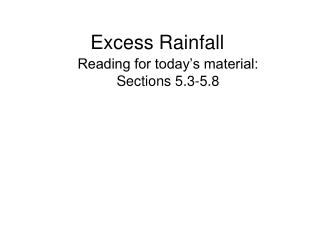DownloadDownload PresentationExcess Rainfall

Excess Rainfall

Download PresentationExcess Rainfall

- - - - - - - - - - - - - - - - - - - - - - - - - - - E N D - - - - - - - - - - - - - - - - - - - - - - - - - - -
Presentation Transcript

1. Excess Rainfall Reading for today’s material: Sections 5.3-5.8

2. Excess rainfall • Rainfall that is neither retained on the land surface nor infiltrated into the soil • Graph of excess rainfall versus time is called excess rainfall hyetograph • Direct runoff = observed streamflow - baseflow • Excess rainfall = observed rainfall - abstractions • Abstractions/losses – difference between total rainfall hyetograph and excess rainfall hyetograph

3. f-index • f-index: Constant rate of abstraction yielding excess rainfall hyetograph with depth equal to depth of direct runoff • Used to compute excess rainfall hyetograph when observed rainfall and streamflow data are available

4. f-index method • Goal: pick Dt, and adjust value of M to satisfy the equation • Steps • Estimate baseflow • DRH = streamflow hydrograph – baseflow • Compute rd, rd = Vd/watershed area • Adjust M until you get a satisfactory value of f • ERH = Rm - fDt

5. Example Have precipitation and streamflow data, need to estimate losses Time Observed Rain Flow in cfs 8:30 203 9:00 0.15 246 9:30 0.26 283 10:00 1.33 828 10:30 2.2 2323 11:00 0.2 5697 11:30 0.09 9531 12:00 11025 12:30 8234 1:00 4321 1:30 2246 2:00 1802 2:30 1230 3:00 713 3:30 394 4:00 354 4:30 303 No direct runoff until after 9:30 And little precip after 11:00 Basin area A = 7.03 mi2

6. Example (Cont.) • Estimate baseflow (straight line method) • Constant = 400 cfs baseflow

7. Example (Cont.) • Calculate Direct Runoff Hydrograph • Subtract 400 cfs Total = 43,550 cfs

8. Example (Cont.) • Compute volume of direct runoff • Compute depth of direct runoff

9. Example (Cont.) • Neglect all precipitation intervals that occur before the onset of direct runoff (before 9:30) • Select Rm as the precipitation values in the 1.5 hour period from 10:00 – 11:30

10. Example (Cont.) fDt=0.27

11. Precipitation Time SCS method • Soil conservation service (SCS) method is an experimentally derived method to determine rainfall excess using information about soils, vegetative cover, hydrologic condition and antecedent moisture conditions • The method is based on the simple relationship that Pe = P - Fa – Ia Pe is runoff depth, P is precipitation depth, Fa is continuing abstraction, and Ia is the sum of initial losses (depression storage, interception, ET)

12. Precipitation Time Abstractions – SCS Method • In general • After runoff begins • Potential runoff • SCS Assumption • Combining SCS assumption with P=Pe+Ia+Fa

13. SCS Method (Cont.) • Surface • Impervious: CN = 100 • Natural: CN < 100 • Experiments showed • So

14. SCS Method (Cont.) • S and CN depend on antecedent rainfall conditions • Normal conditions, AMC(II) • Dry conditions, AMC(I) • Wet conditions, AMC(III)

15. SCS Method (Cont.) • SCS Curve Numbers depend on soil conditions

16. Example - SCS Method - 1 • Rainfall: 5 in. • Area: 1000-ac • Soils: • Class B: 50% • Class C: 50% • Antecedent moisture: AMC(II) • Land use • Residential • 40% with 30% impervious cover • 12% with 65% impervious cover • Paved roads: 18% with curbs and storm sewers • Open land: 16% • 50% fair grass cover • 50% good grass cover • Parking lots, etc.: 14%

17. Example (SCS Method – 1, Cont.) CN values come from Table 5.5.2

18. Example (SCS Method – 1 Cont.) • Average AMC • Wet AMC

19. Example (SCS Method – 2) • Given P, CN = 80, AMC(II) • Find: Cumulative abstractions and excess rainfall hyetograph

20. Example (SCS Method – 2) • Calculate storage • Calculate initial abstraction • Initial abstraction removes • 0.2 in. in 1st period (all the precip) • 0.3 in. in the 2nd period (only part of the precip) • Calculate continuing abstraction

21. Example (SCS method – 2) • Cumulative abstractions can now be calculated

22. Example (SCS method – 2) • Cumulative excess rainfall can now be calculated • Excess Rainfall Hyetograph can be calculated

23. Example (SCS method – 2) • Cumulative excess rainfall can now be calculated • Excess Rainfall Hyetograph can be calculated

24. Time of Concentration • Different areas of a watershed contribute to runoff at different times after precipitation begins • Time of concentration • Time at which all parts of the watershed begin contributing to the runoff from the basin • Time of flow from the farthest point in the watershed Isochrones: boundaries of contributing areas with equal time of flow to the watershed outlet

25. Stream ordering • Quantitative way of studying streams. Developed by Horton and then modified by Strahler. • Each headwater stream is designated as first order stream • When two first order stream combine, they produce second order stream • Only when two streams of the same order combine, the stream order increases by one • When a lower order stream combines with a higher order stream, the higher order is retained in the combined stream